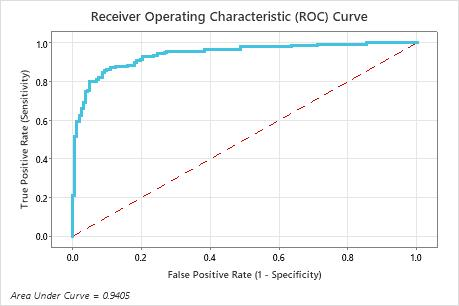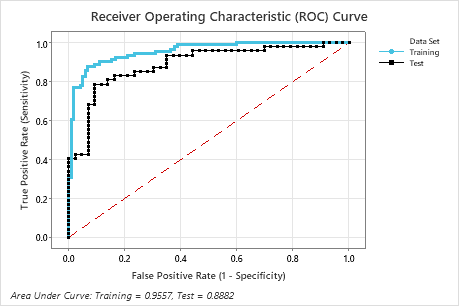# Receiver operating characteristic (ROC) curve for Fit Binary Logistic Model

The ROC curve plots the true positive rate (TPR), also known as power, on the y-axis. The ROC curve plots the false positive rate (FPR), also known as type 1 error, on the x-axis. The area under an ROC curve indicates whether the binary model is a good classifier.

## Interpretation

The area under the ROC curve values range from 0.5 to 1. When the binary model can perfectly separate the classes, then the area under the curve is 1. When the binary model cannot separate the classes better than a random assignment, then the area under the curve is 0.5.

When no separate test set is used, Minitab creates the ROC curve with the data set.In this example, the area under the test curve is 0.9405.

With a test set, Minitab creates two ROC curves. One curve is for the training data and the other is for the test data. The test results indicate whether the model can adequately predict the response values for new observations, or properly summarize the relationships between the response and the predictor variables. The training results are usually more ideal than actual and are for reference only.In this example, the training and test curves are similar to each other. The area under the test curve is 0.8882.
By using this site you agree to the use of cookies for analytics and personalized content.  Read our policy